Goseeko blog# What is binomial distribution?

## Introduction

A distribution is said to be a binomial distribution if the following conditions are met.

1. Each trial has a binary outcome (One of the two outcomes is labeled a ‘success’)
2. The probability of success is known and constant over all trials
3. The number of trials is specified
4. The trials are independent. That is, the outcome from one trial doesn’t affect the outcome of successive trials

If all the above conditions met then the binomial distribution describes the probability of X successes in n trials.

## Binomial distribution

A classic example of the binomial distribution is the number of heads (X) in n coin tosses.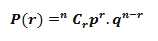To find the probability of the happening of an event once, twice, thrice,…r times ….exactly in n trails.

Let the probability of the happening of an event A in one trial be p and its probability of not happening be 1 – p – q.

We assume that there are n trials and the happening of the event A is r times and its not happening is n – r times.

This may be shown as follows

AA……A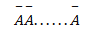r times                                   n – r times                      (1)

A indicates its happening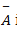its failure and P (A) =p and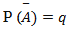We see that (1) has the probability

r times          n-r times                                                       (2)

Clearly (1) is merely one order of arranging r A’S.

These terms are clearly the successive terms in the expansion of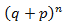Hence it is called Binomial Distribution.

## Solved examples

Example. If on an average one ship in every ten is wrecked. Find the probability that out of 5 ships expected to arrive, 4 at least we will arrive safely.

Solution:

Out of 10 ships one ship is wrecked.

I.e. nine ships out of 10 ships are safe, P (safety) = 9/10

P (at least 4 ships out of 5 are safe) = P (4 or 5) = P (4) + P(5)

Example. The overall percentage of failures in a certain examination is 20. if 6 candidates appear in the examination what is the probability that at least five pass the examination?

Solution:

Probability of failures = 20% = 20/100 = 1/5

(P) = 1- ⅕ = 4/5

Probability of at least 5 pass = P(5 or 6)

Example:

The probability that a man aged 60 will live to be 70 is 0.65. what is the probability that out of 10 men, now 60, at least seven will live to be 70?

Solution:

The probability that a man aged 60 will live to be 70 = p = 0.65

q = 1 – 0.65 = 0.35

Number of men= n = 10

Probability that at least 7 men will live to 70 = (7 or 8 or 9 or 10)

Example

Assuming that 20% of the population of a city are literate so that the chance of an individual being literate is 1/5 and assuming that hundred investigators each take 10 individuals to see whether they are illiterate, how many investigators would you expect to report 3 or less were literate.

Solution

Required number of investigators = 0.879126118× 100 =87.9126118 =  = 88 approximate